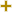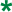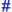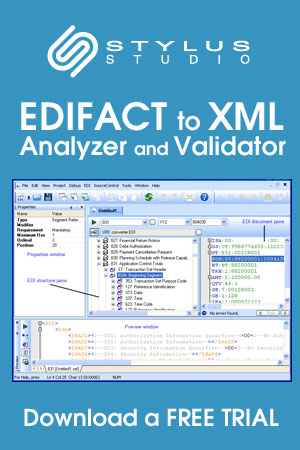Return to Stylus Studio EDIFACT D97B Code List page.

# Simple data element character representation, codedUN/CEFACT Revision 1997B Code ListRepr:an..3
Desc:To specify the character representation of a simple data element.
This table also occurs in the following versions of this standard:
D00A, D00B, D01A, D01B, D01C, D02A, D02B, D03A, D03B, D04A, D04B, D95B, D96A, D96B, D97A, D97B, D98A, D98B, D99A, D99B

 1 Alphabetic Simple data element character type is alphabetic. 2 Alphanumeric Simple data element character type is alphanumeric. 3 Numeric Simple data element character type is numeric. 4 Binary Simple data element character type is binary. 5 Date Simple data element character type is a date. 6 Identifier Simple data element character type is an identifier. 7 Time Simple data element character type is time. 8 Numeric, implied decimal point of 0 places Simple data element character type is numeric with an implied decimal point of 0 places as determined from right to left. 9 String Simple data element character type is a string. 10 Numeric, implied decimal point of 1 place Simple data element character type is numeric with an implied decimal point of 1 place as determined from right to left. 11 Numeric, implied decimal point of 2 places Simple data element character type is numeric with an implied decimal point of 2 places as determined from right to left. 12 Numeric, implied decimal point of 3 places Simple data element character type is numeric with an implied decimal point of 3 places as determined from right to left. 13 Numeric, implied decimal point of 4 places Simple data element character type is numeric with an implied decimal point of 4 places as determined from right to left. 14 Numeric, implied decimal point of 5 places Simple data element character type is numeric with an implied decimal point of 5 places as determined from right to left. 15 Numeric, implied decimal point of 6 places Simple data element character type is numeric with an implied decimal point of 6 places as determined from right to left. 16 Numeric, implied decimal point of 7 places Simple data element character type is numeric with an implied decimal point of 7 places as determined from right to left. 17 Numeric, implied decimal point of 8 places Simple data element character type is numeric with an implied decimal point of 8 places as determined from right to left. 18 Numeric, implied decimal point of 9 places Simple data element character type is numeric with an implied decimal point of 9 places as determined from right to left.

Change indicators
plus signAn addition.
asteriskAddition/substraction/change to a code entry for a particular data element.
hash or pound signChanges to names.
vertical barChanges to text for descriptions, notes and functions.
minus signA deletion.
letter XMarked for deletion.

Usage indicatorsUsed in batch messages only.Common usage in both batch and interactive messages.Used in interactive messages only.

Return to Stylus Studio EDIFACT D97B Code List page.